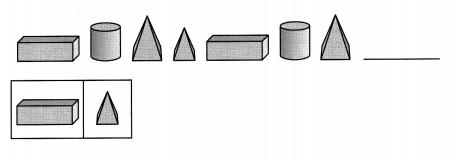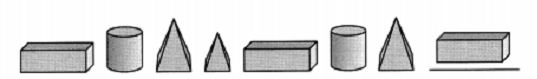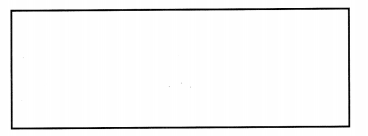# Math in Focus Grade 1 Chapter 5 Answer Key Shapes and Patterns

This handy Math in Focus Grade 1 Workbook Answer Key Chapter 5 Shapes and Patterns detailed solutions for the textbook questions.

## Math in Focus Grade 1 Chapter 5 Answer Key Shapes and Patterns

Math Journal

Choose two things. Circle them.

Question 1.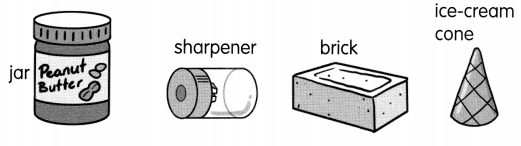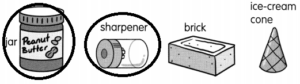cylinder sphere cube cone pyramid rectangular prism stacking sliding rolling size shape

Question 2.
The ____________ has the shape of a ____________
Answer: The jar has the shape of a cylinder.

Question 3.
The ______________ has the shape of a ____________
Answer: The sharpener has the shape of a cylinder.

Question 4.
I can move the ____ by ____________
Answer: I can move the cylinder by rolling

Question 5.
I can move the ____ by ____________
Answer: I can move the sphere by sliding

Question 6.
My things are alike because they ____________
Answer: My things are alike because they are different in size.

Question 7.
My things are different because they ____
Answer: My things are different because they are different in shape.

Make a pattern with plane shapes. Read and draw.

Question 8.
The shapes in this pattern are alike. The sizes of the shapes are different.
_________________________

challenging Practice

Solve.

Question 1.
Lee, Jen, Bob, and Dean have some shapes. Find out who has each set of shapes.

• Lee has fewer circles than Bob.
• All of Jen’s shapes have 3 sides or more.
• Bob has four kinds of shapes.
• Dean has no squares.

Write the name that matches each set on the line below.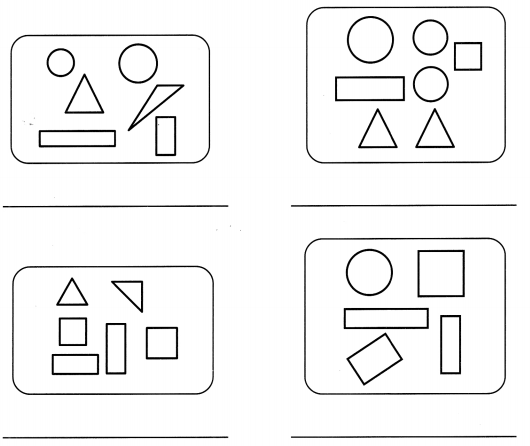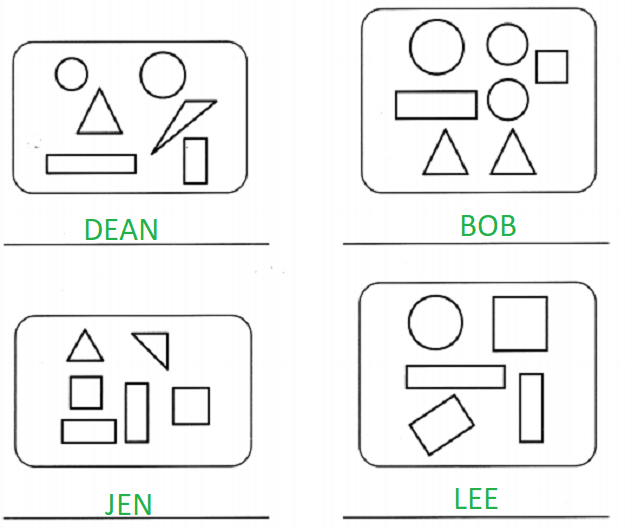Cut out the pieces of shapes on page 129. Paste the cut-out pieces to fit the two pictures below.

Question 2.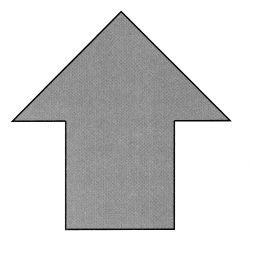Question 3.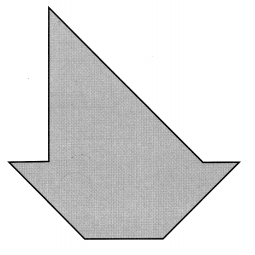Question 4.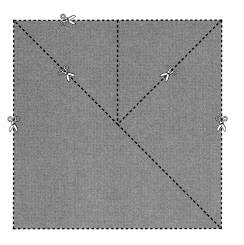Question 5.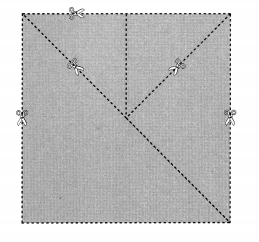Problem Solving

Draw and complete the pattern.

Each row (↔) and column (↕) must have these four shapes,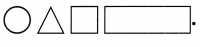Question 7.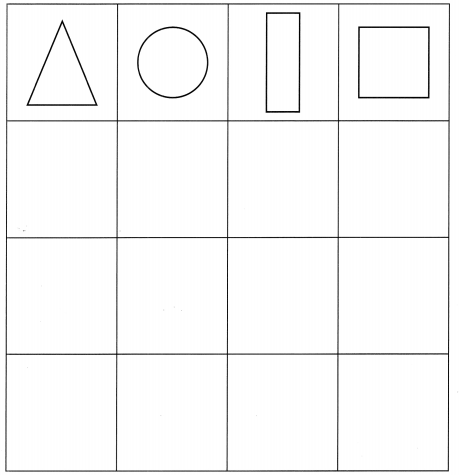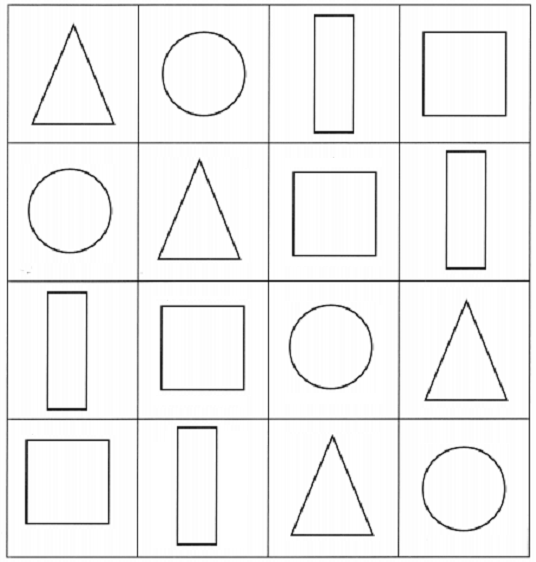Chapter Review/Test

Vocabulary

Draw the shape.

Question 1.
square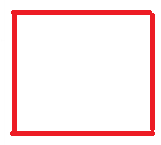Question 2.
rectangle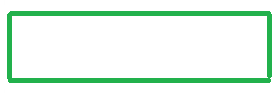Question 3.
triangle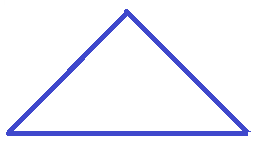Write the name.
Use the words in the box.

Question 4.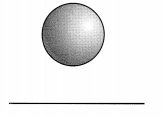Question 5.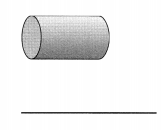Concepts and Skills
Trace the shape.
Write the number of sides and corners.

Question 6.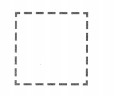___ sides
___ corners
Answer: Square has 4 sides and 4 corners.

Question 7.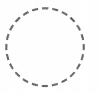___ sides
___ corners
Answer: Circle has 0 sides and 0 corners.

Answer the question. Write yes or no.

Question 8.
How are these shapes alike?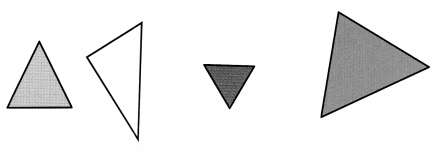a. Same shape ____
Answer: Figure 1 and figure 3 has the same shape

b. same size _______

c. same color ________

Circle the solid shapes you can roll.

Question 9.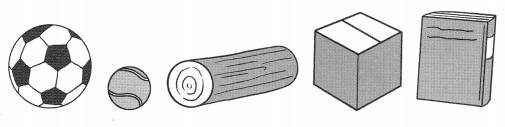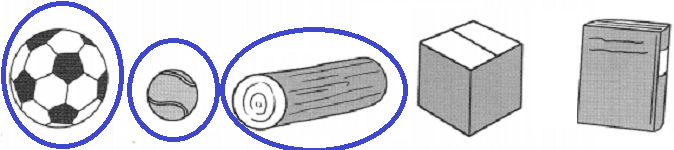How can you move a pyramid? Circle the answer.

Question 10.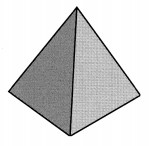stack
slide
roll
Answer: Pyramid can be moved by stacking.

Look at the picture. What shapes do you see? Write the number.

Question 11.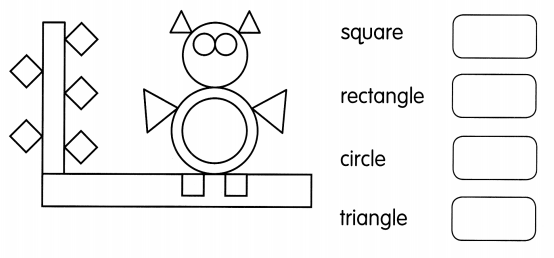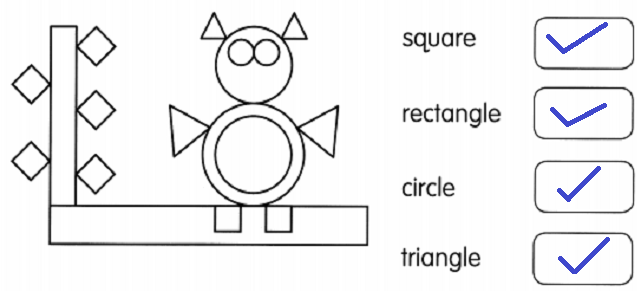Look at the picture. What shapes do you see? Circle the answers.

Question 12.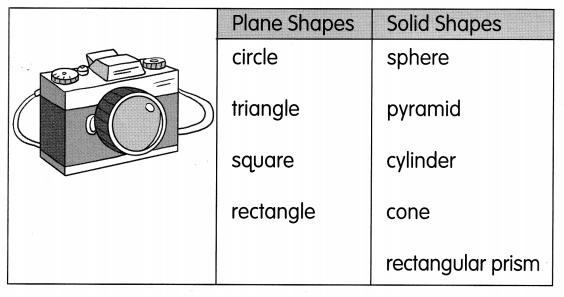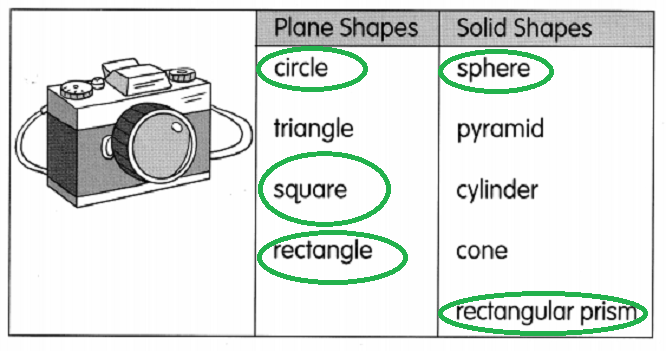Complete the pattern. Circle the shape that comes next.

Question 13.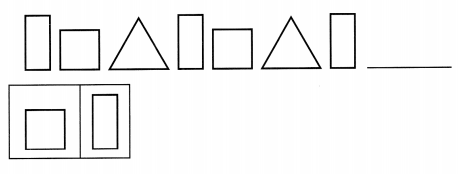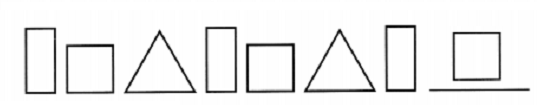Question 14.